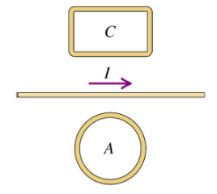# Problem: Two closed loops A and C are close to a close wire carrying a current(a) Find the direction (clockwise or counterclockwise) of the current induced in the loop A if I is steadily increasinga. The current in loop A is counterclockwiseb. The current in loop A is clockwisec. The current in loop A is zero(b) While I is increasing, what is the direction of the net force that the wire exerts on loop A?a. The wire exerts an upward force on loop Ab. The wire exerts a downward force on loop Ac. The wire exerts no force on loop A

###### FREE Expert Solution

Right-hand rule for straight conductors: Thumb points in the direction of current, fingers curl in the direction of magnetic field.

Right-hand rule for lops: Thumb points in direction of the magnetic field. Fingers curl in the direction of the current.

Lenz's law:

The induced current creates an induced magnetic field that opposes the change in magnetic flux inducing it.

(a)

The field due to the wire carrying a current in loop A is into the page. The change in flux is increasing.

The induced field is out of the page to oppose the increasing flux.

92% (33 ratings)###### Problem Details

Two closed loops A and C are close to a close wire carrying a current(a) Find the direction (clockwise or counterclockwise) of the current induced in the loop A if I is steadily increasing

a. The current in loop A is counterclockwise

b. The current in loop A is clockwise

c. The current in loop A is zero

(b) While I is increasing, what is the direction of the net force that the wire exerts on loop A?

a. The wire exerts an upward force on loop A

b. The wire exerts a downward force on loop A

c. The wire exerts no force on loop A